#PQStat - Baza Wiedzy

### Pasek boczny

en:statpqpl:aopisowapl:tabliczpl

# Tables

## Frequency tables and empirical distribution of the data

The basis of statistical research is the determination of the empirical distribution, i.e., the distribution of a feature observed in a sample. The empirical distribution is determined by assigning a frequency of occurrence to successive values of the feature. Such distribution can be presented in the form of frequency table or as a graph (histogram). For small data sets, frequency tables can present all data - the so-called point distribution series, while for larger data sets the so-called interval distribution series are created.

To represent the data distribution in table form, bring up the Frequency tables window by selecting menu StatisticsDescriptive analysisFrequency tables.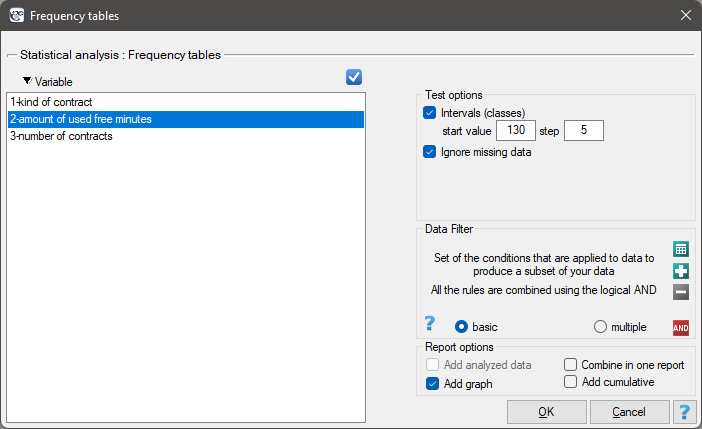In this window we choose a variable to analyse and options for analysis. You can sort the output as a text or as a number by selecting the appropriate options. If there are empty cells in the analysed column, they may be included or omitted in the analysis. The result of analysis will be placed in report attached to datasheet, for which analysis has been done.

In addition, if you want the data to be visualized with a column chart or histogram, then in the Frequency table window, check the Add graph option..

EXAMPLE (distribution.pqs file)

A mobile operator conducts a series of surveys on how customers use the number of „free minutes” they are given in their subscription. Customers can use up to 190 such minutes each month. The study was based on a random sample of 200 customers. Information analysed included:

- type of subscription purchased,

- number of free minutes used,

- number of subscriptions registered for a given customer (does not apply to companies).

We want to present the distribution of:

1. type of subscription,
2. number of free minutes used,
3. number of registered subscriptions for an individual person.

Open the Frequency table window..

1. Select the Variable to analyse: „type of subscription” and Add graph. Then confirm the selected settings with OK button and the result is obtained as a report: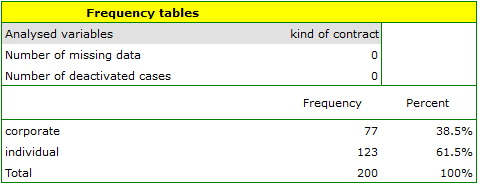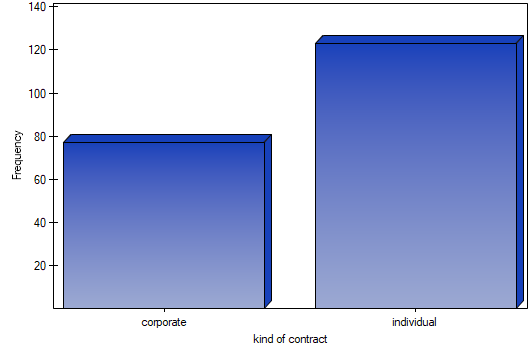1. Resume Analysis by pressing. We select the variable to analyse: „amount of used free minutes” and check the option Intervals (classes), set start value for example to 130 and step to 5. We can also check the option Add graph. Then confirm the selected options with OK and the result is obtained as a report: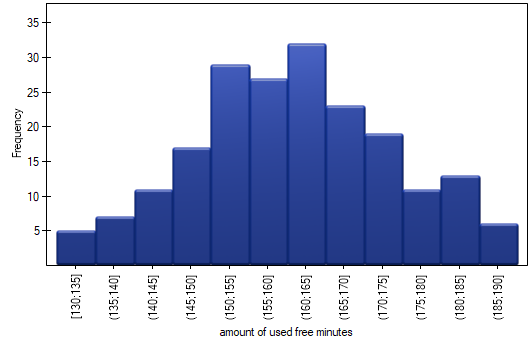1. Resume Analysis by pressing. We set filter so that the analysis is performed only for individuals. We select the variable to analyse: „Number of subscriptions”. Since this variable also contains missing data, the result obtained may or may not include these missing cases in the analysis, depending on the option selected: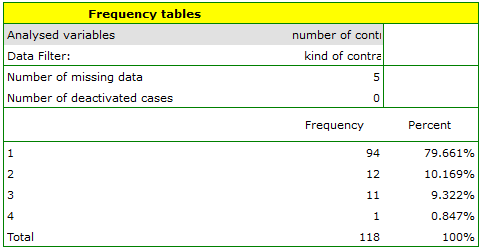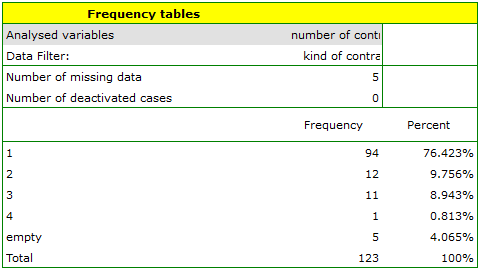EXAMPLE (fertiliser.pqs file)

An experiment was conducted to study the microbiological condition of soil under perennial ryegrass cultivation supplied with biologically active fertilizers. Soils were fertilized with different types of microbial preparations and fertilizers and then the number of microorganisms present per gram of soil dry matter was calculated. We want to know the frequency of actinomycetes per 1 gram of dry nitrogen fertilized soil. We are interested in how often 0 to 20 actinomycetes were present in the sample, more than 20 to 40 actinomycetes, more than 40 to 60 actinomycetes, etc. We select only the first 54 rows in the datasheet that match the assumptions of the analysis (these are nitrogen-fertilized actinomycetes) and open the Frequency Tables.

In the options window, we select the variable to be analysed: Number of microorganisms, and then set the class intervals so that the start value is 0 and the step is 20. You should see a message at the top of the window: \textcolor{black}{

Data limited by selection

. Confirm the selection with the OK button and the result should appear as a report: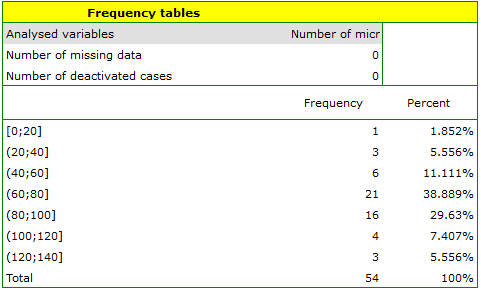2022/02/09 12:56

## Table report

Using a table report, you can prepare a simultaneous summary of a large amount of data in the form of bivariate tables (tables of two features). For example, we can present the distribution of age groups by place of residence, education, etc. in the form of a table. Each table is presented in the form of frequency in particular categories, and additionally, it can be summarized by calculating percentages from a row, from a column, or from the total sum, and determining the frequency table expected. In addition, automatic summaries in the form of a column chart are possible for such tables. The window with the table report settings is opened via menu StatisticsDescriptive analysisTable report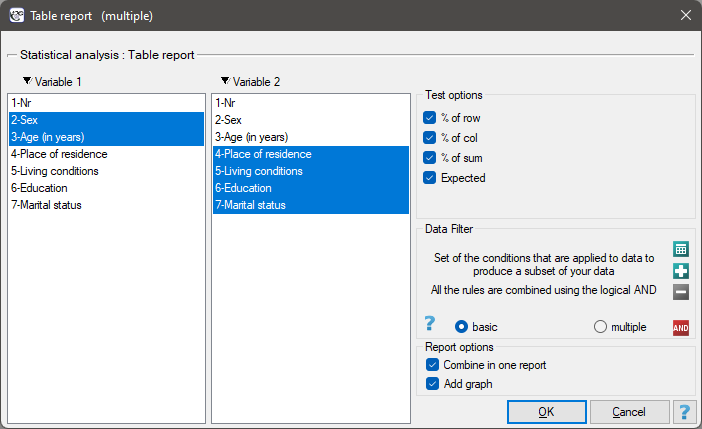EXAMPLE (Tables.pqs file)

In the form of tables, we need to summarize the distribution of gender by place of residence, social and living conditions, education, marital status, and the distribution of age groups with respect to the same characteristics. This will result in 4 tables for each pair of traits, or 8 tables for all pairs and corresponding graphs. Only the distribution with respect to gender is presented below: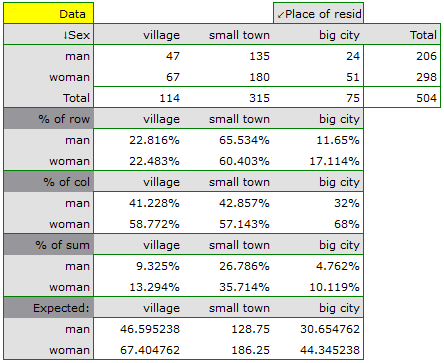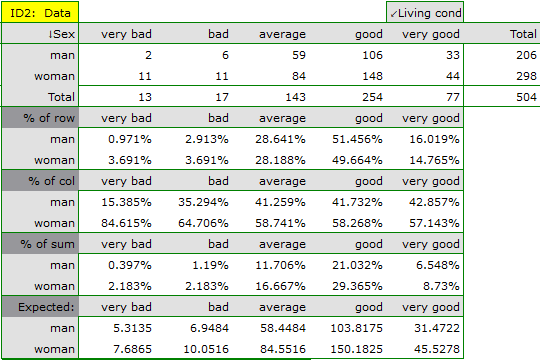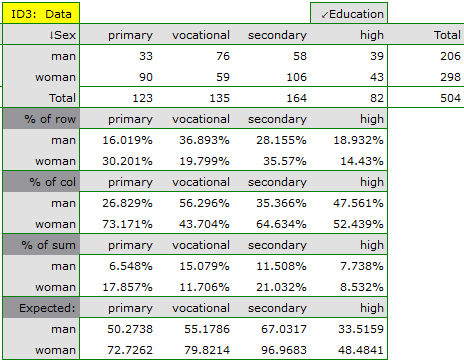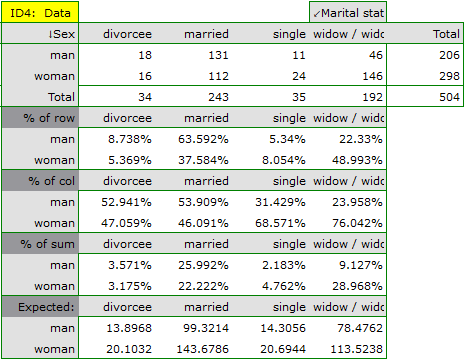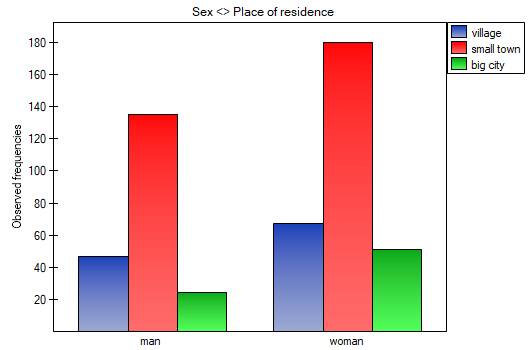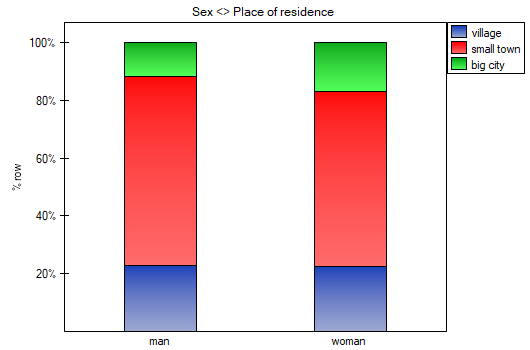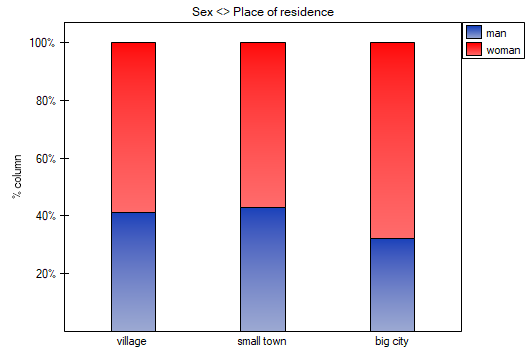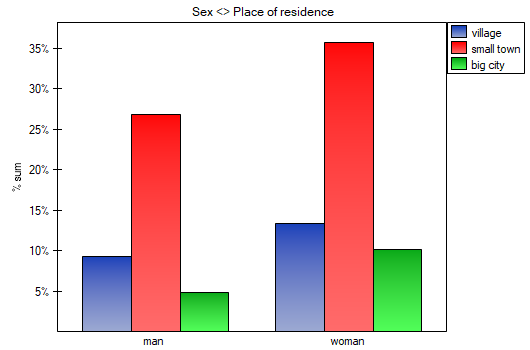For the distribution with respect to age groups, age categories were first created through codes/labels/format.

2022/02/09 12:56

## Analyses for contingency tables

Analyses for the contingency tables can be computed from data collected in the contingency tables or directly i.e., from raw data. Whereby it is possible to transform the data from the contingency table to the raw form or vice versa.

EXAMPLE (sex-education.pqs file)

Consider a sample consisting of 34 individuals (). We examine 2 traits of these individuals (=sex,=education). Gender appears in 2 categories (=female,=male) education in 3 categories, (=primary + vocational=medium,=higher).

In the case of raw data, when you open the test options window, e.g., thefor thetables, the raw data option will automatically be selected..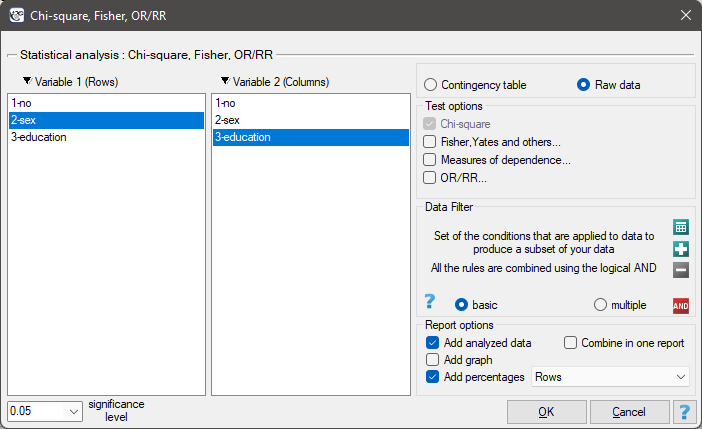For data collected in a contingency table, it is a good idea to select this data (numerical values without headers) before opening the test window. Then, when you open the test window, the contingency table option will automatically be selected and the data from the selection will be displayed.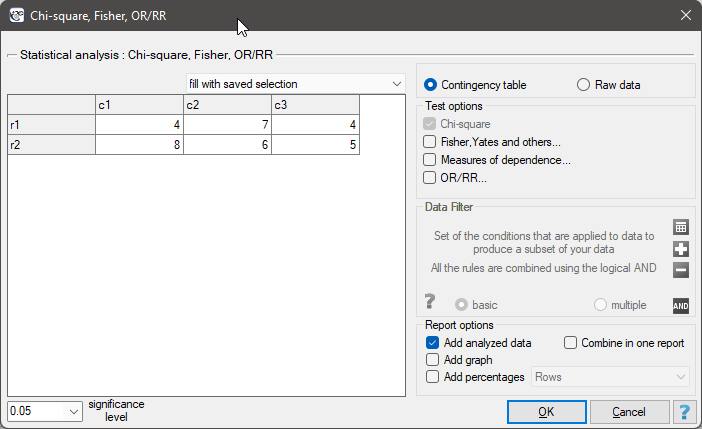In the test window, we can always change the automatically detected setting regarding the form of data organization, as well as enter data into the contingency table from the window.

Cochran's condition

This is a basic condition for using many statistical tests based on contingency tables, e.g., the chi-square test. This condition implies a large expectred frequencies. According to Cochran's 1952 interpretation1), none of the expected frequencies can beand no more than 20% can be. Information about whether this condition is met (or not) by the data collected in the table can be returned to the report.

Basic tests for contingency tables:

Coefficients for contingency tables:

You can also include a basic summary of the tables in the results report:

• Contingency table of observed frequenciesthat is, data in the form of a contingency table. Such a table shows the distribution of observations for several traits (several variables). Table for 2 traits (,), of which the first has possibleand the secondcategories are shown below).Frequencies observed() represent the frequency of each category for both traits.

In order for such a table to be returned by the program, the option include analysed data should be selected in the test window. For the data from the example, the contingency table of observed frequencies is as follows: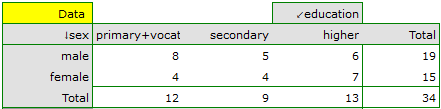• A contingency table of expected frequenciesfor each contingency table of observed frequencies, a corresponding table of expected frequencies:can be createdwhere:,,,,,,.

For the data in the example The contingency table of expected frequencies is as follows: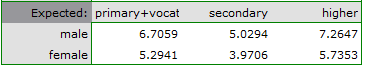• Contingency table of percentages calculated from the sum of columns. For the data in the example this table is as follows: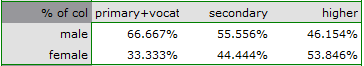• Tcontingency table of percentages calculated from the sum of the rows. For the data in the example this table is as follows: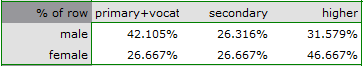• A contingency table of percentages calculated from the sum of the total rows and columns. For the data in the example this table is as follows: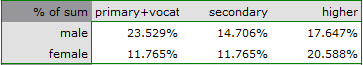2022/02/09 12:56
1)
Cochran W.G. (1952), The chi-square goodness-of-fit test. Annals of Mathematical Statistics, 23, 315-345
en/statpqpl/aopisowapl/tabliczpl.txt · ostatnio zmienione: 2022/03/14 11:25 przez admin

### Narzędzia strony